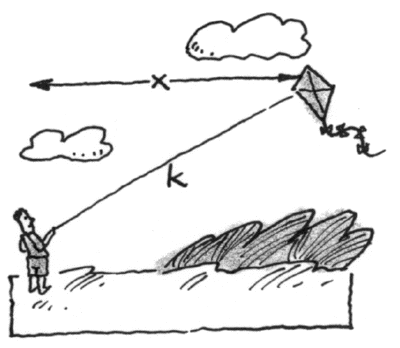### Home > CALC > Chapter 7 > Lesson 7.1.1 > Problem7-12

7-12.

As the length, $k$, of rope changes, the kite flies horizontally either toward the person or away from the person. (The altitude of the kite remains constant). Are $\frac { d k } { d t }$ and $\frac { d x } { d t }$ positive or negative? Describe the way $\frac { d x } { d t }$ changes.Both $\frac{dk}{dt}$ and $\frac{dx}{dt}$ are positive.